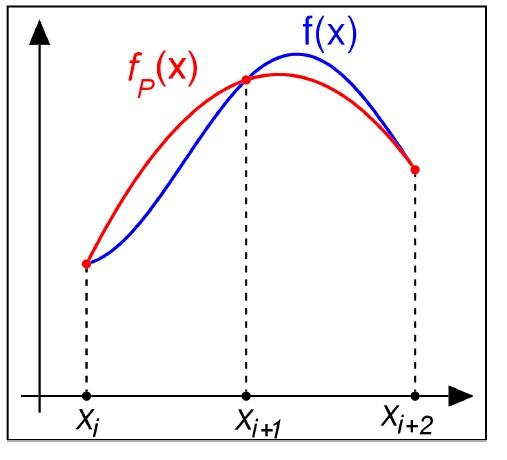### ACM 20030 Computational ScienceI do not currently teach this module. The materials on this page are outdated and are left here for archival purposes only.

Description: Typically, problems in Applied Mathematics are modelled using a set of equations that can be written down but cannot be solved analytically. In this module we examine numerical methods that can be used to solve such problems on a desktop computer. Practical computer lab sessions will cover the implementation of these methods using mathematical software (Matlab). No previous knowledge of computing is assumed.

Topics and techniques discussed include but are not limited to the following list:

• Computer architecture: The Von Neumann model of a computer, memory hierarchies, the compiler.
• Floatingpoint representation: Binary and decimal notation, floating-point arithmetic, the IEEE double precision standard, rounding error.
• Elementary programming constructions: Loops, logical statements, precedence, array operations, vectorization.
• Root-finding for single-variable functions: Bracketing and Bisection, Newton-Raphson method. Error and reliability analyses for the Newton-Raphson method.
• Numerical integration: Midpoint, Trapezoidal and Simpson methods. Error analysis.
• Solving ordinary differential equations (ODEs): Euler Method, Runge-Kutta method. Stability and accuracy for the Euler method.
• Linear systems of equations: Gaussian elimination, partial pivoting.
• The condition number of a matrix: quantifying the idea that a matrix can be "almost" singular, investigating the consequences of this idea for the robustness of numerical solutions of linear systems.
• Fitting data to polynomials using the method of least squares.
• Random-number generation using the linear congruential method.

Learning Outcomes: On completion of this module students should be able to:

1. Describe the architecture of a modern computer using the Von Neumann model.
2. Describe how numbers are represented on a computer.
3. Use floating-point arithmetic, having due regard for rouding error.
4. Do elementary operations in Matlab, such "for" and "while" loops, logical statements, precdence.
5. Do array operations using loops; and equivalently, using vectorization.
6. Describe elementary root-finding procedures, analyse their robustness, and implement them in Matlab.
7. Describe elementary numerical integration integration schemes, analyse their accuracy, and implement them in Matlab.
8. Solve ODEs numerically uzing standard algorithms, analyse their accuracy and stability, and implement them numerically.
9. Solve systems of linear equations using Gaussian elimination.
10. Analyse ill-conditioned systems of equations.
11. Fit data to polynomials.

Course documents: I no longer teach this module. However, a complete set of lecture notes relating to an earlier edition of the course (2013-2014) is available here.